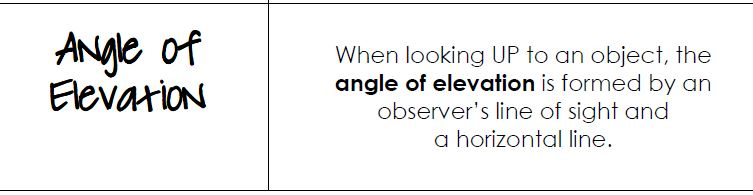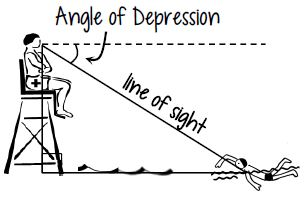Right Triangle Trigonometry in Real Life
starstarstarstarstarstarstarstarstarstar
5 (2 ratings)
by Robyn Porter
| 15 Questions
In this lesson, you will apply what you know about solving right triangles to real-life situations.

Before you proceed, you should know the basics of Trigonometry, like how to:
- Label sides of the triangle (hypotenue, opposite, adjacent)
- Choose the right ratio (sine, cosine, or tangent)
- Use the Pythagorean Theorem to find a missing side
- Use the Triangle Sum Theorem to find a missing angle
- Solve an equation involving trig (find the missing side or angle)

If you'd like more practice on any of these foundational skills, please go back to the hyperdoc and select the Google Form in the "Remediate" section.

# VOCABULARY1
1
The "line of sight" is what part of the right triangle?
A - Opposite
C - Hypotenuse2
1
The angle of depression is ____________ to the angle of elevation because they are _______________________.
A - complementary; corresponding angles
B - congruent; corresponding angles
C - complementary; alternate interior angles
D - congruent; alternate interior angles

# Tips for solving application problems:

• Mark the right angle in the diagram.
• Mark given angle measures or side lengths.
• Use “x” to mark the side/angle you're asked to find.
• Decide which trig function to use.
• Write an equation and solve.

# Let's Practice!

3
1
Draw the variable x on the side or angle that is described below.
4
1
Draw the variable x on the side or angle that is described below.
Once you place the variable in the correct place, you can determine which trig ratio to use. Give it a try.
5
1
Label the picture with the given information and place an x on the part we want to know.
6
1
Which trig ratio can be used to solve this problem?
A - sin
B - cos
C - tan
7
1
What is the approximate height of the tower? Round to the nearest tenth. (numberonly)
8
1
Label the picture with the given information and place an x on the part we want to know.
9
1
Which trig ratio can be used to solve this problem?
A - sin
B - cos
C - tan
10
1
What is the approximate height of the lamp post? Round to the nearest tenth. (number only)
11
1
Label the picture with the given information and place an x on the part we want to know.
12
1
Which trig ratio can be used to solve this problem?
A - sin
B - cos
C - tan
Was that last problem tricky?
The phrase "how much farther does the plane have to fly before it crosses the coast" describes the horizontal distance. Did you mark the horizontal with an "x"?

13
1
Label the picture with the given information and place an x on the part we want to know.
14
1
Which trig ratio can be used to solve this problem?
A - sin
B - cos
C - tan
15
1
What is the approximate measure of the angle to the nearest degree? (number only)
This concludes the learning module. Be sure to review your answers and ask questions if you're not sure why you got one wrong (if applicable). Now, you should return to the hyperdoc for additional instructions.